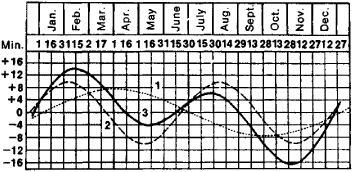# equation of time

(redirected from equations of time)
Also found in: Dictionary.

## equation of time:

see solar timesolar time,
time defined by the position of the sun. The solar day is the time it takes for the sun to return to the same meridian in the sky. Local solar time is measured by a sundial.
.

## equation of time

The correction that must be applied to apparent solar time to obtain mean solar time, i.e. it is the difference in time as measured by a sundial and by a clock. The equation of time varies through the year; it has two maxima and two minima and is zero on four dates: April 15/16, June 14/15, Sept. 1/2, and Dec. 25/26. A positive value indicates that apparent time is ahead of mean time; the greatest positive value, which can be over 16 minutes, occurs in early Nov.; the greatest negative value, over 14 minutes, occurs in mid-Feb. The curve is the sum of two components, each reflecting a nonuniformity in the apparent motion of the Sun: one component arises from the ellipticity of the Earth's orbit, the other from the inclination of the ecliptic to the celestial equator (see mean Sun).
Collins Dictionary of Astronomy © Market House Books Ltd, 2006
The following article is from The Great Soviet Encyclopedia (1979). It might be outdated or ideologically biased.

## Equation of Time

the difference between mean solar time and apparent solar time, equal to the difference between the right ascensions of the true sun and the mean sun. The equation of time is often defined as the difference between apparent time and mean time; in this case, the equation has the opposite sign, a fact that must be borne in mind when reference books are used.

The equation of time varies continuously. The variation is due to the fact that the passage of apparent solar time, as measured by the hour angle of the true sun, is nonuniform because of the nonuniformity of the earth’s orbital motion and the inclination of the ecliptic to the equator. Therefore, the equation of time is obtained by adding two waves of approximately sinusoidal shape and nearly equal amplitude (see Figure 1). One of the waves has an annual period; the other, a semiannual period. The equation of time is equal to zero four times a year—around Apr. 16, June 14, Sept. 1, and Dec. 25. Four times a year it attains the following largest absolute values: +14.3 min (around Feb. 12), –3.8 min (around May 15), +6.4 min (around July 27), and –16.4 min (around Nov. 4).Figure 1. Plot of the equation of time: (1) component of the equation determined by the nonuniformity of the earth’s orbital motion, (2) component of the equation determined by the Inclination of the ecliptic to the equator, (3) the equation of time

We can find the local mean solar time by using the equation of time if we know the apparent solar time, as determined from observations of the sun with, for example, a sundial. In this case, we use the following formula:

m = m0 + η

where m is the mean time, m0 is the apparent time, and η in is the equation of time.

Values of the equation of time for each day are given in astronomical almanacs and calendars. (See.)

## equation of time

[i′kwā·zhən əv ′tīm]
(astronomy)
The addition of a quantity to mean solar time to obtain apparent solar time; formerly, when apparent solar time was in common use, the opposite convention was used; apparent solar time has annual variation as a result of the sun's inclination in the ecliptic and the eccentricity of the earth's elliptical orbit.
McGraw-Hill Dictionary of Scientific & Technical Terms, 6E, Copyright © 2003 by The McGraw-Hill Companies, Inc.

## equation of time

The amount of time by which the mean sun leads or lags behind the true sun at any instant. Its value is positive or negative, depending on whether the true sun is ahead of or behind the mean sun. Its value never exceeds 16¼ min. It is the correction applied to 12 h + local mean time to obtain the local hour angle of the sun.